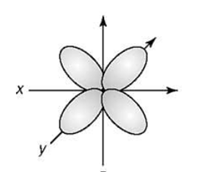# Problem: Based on the following atomic orbital shape, which of the following set of quantum numbers is correct:  a) n = 3, ℓ = 2, m ℓ = 0, m s = –1/2 b) n = 3, ℓ = 1, m ℓ = –3 , m s =  1 c) n = 4, ℓ = 0, m ℓ = 0, m s = –1/2 d) n = 4, ℓ = 2, m ℓ​ = –3, m s = –1/2

###### FREE Expert Solution
89% (321 ratings)
###### Problem Details

Based on the following atomic orbital shape, which of the following set of quantum numbers is correct:

a) n = 3, ℓ = 2, m  = 0, m s = –1/2

b) n = 3, ℓ = 1, m  = –3 , m s =  1

c) n = 4, ℓ = 0, m  = 0, m s = –1/2

d) n = 4, ℓ = 2, m ℓ​ = –3, m s = –1/2What scientific concept do you need to know in order to solve this problem?

Our tutors have indicated that to solve this problem you will need to apply the Quantum Numbers: Magnetic Quantum Number concept. You can view video lessons to learn Quantum Numbers: Magnetic Quantum Number. Or if you need more Quantum Numbers: Magnetic Quantum Number practice, you can also practice Quantum Numbers: Magnetic Quantum Number practice problems.

What is the difficulty of this problem?

Our tutors rated the difficulty ofBased on the following atomic orbital shape, which of the fo...as medium difficulty.

How long does this problem take to solve?

Our expert Chemistry tutor, Jules took 3 minutes and 25 seconds to solve this problem. You can follow their steps in the video explanation above.

What professor is this problem relevant for?

Based on our data, we think this problem is relevant for Professor Saber's class at TAMU.